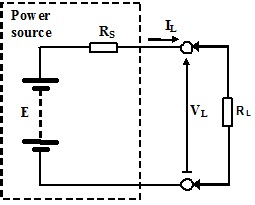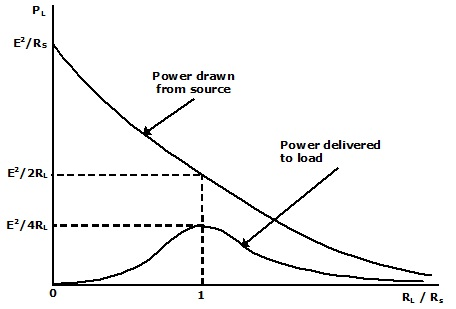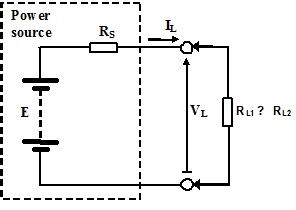#### Theory of Load Power and Internal Resistance

Introduction:

Even in the easiest of electric circuits the ultimate goal is generally to deliver power to a load. In circuits we have observed to date, the load has been modelled as a pure resistance, RL. Though, the load is not for eternity a real resistor however the resistor is employed to symbolize any load, operation or process that used up electrical energy and thus power. In reality, the simplified electric circuits can themselves be models for power sources, equipment or instruments that give electrical energy to a load. If this is the situation, then the factors that influence the transfer of power to the load from the source must be examined to make sure the optimum use of the energy consumed.

Maximum Power Transfer:

The circuit shown in figure below can be considered as a model symbolizing a non-ideal source that has an internal resistance, RS, driving a load resistance, RL. The power dissipated in the load is provided as:
PL = VLIL

Where VL is the voltage appearing across load and IL is the current flowing via it.Figure: Power Source driving a Resistive Load

The voltage across the load is basically obtained from the potential divider action of RS and RL that offers:

VL = [(RL)/(RS +RL)]E

The current via the load is essentially received from the source emf divided by the sum of two resistors in series.

IL = E/(RS + RL)

Then:

PL = {ERL/(RS + RL)}{E/(RS + RL)} = (E2RL)/(RS + RL)2

This can be seen that if the voltage source is ideal with RS → 0, then the power would be:

PL = (E2RL)/RL = E2/RL

In this event, the full emf, E, is build up across the load resistance, RL, and power delivered to the load depends only the value of this emf and the value of load resistance. This signifies that all the power drawn from source is dissipated in the load resistance, RL.

On other hand, whenever the source is non-ideal and RS is finite, some power will be dissipated in this resistance and is thus essentially ‘wasted’ or ‘lost’. The remainder of power is delivered to the load as:

PL = (E2RL)/(RS +RL)2

Generally, the internal resistance of the source or equivalent model symbolizing the source is determined by the factors beyond control and therefore can’t always be adjusted to suit requirements. Though, the resistance of the load can frequently be controlled by design, at least to certain degree.

In this case, the goal is to try to optimise the load resistance, RL, and hence maximum power is transferred from the source to load. This can be found out by finding the derivative of the expression for load power in respect to the load resistance, RL and equating it to zero. Then if:

PL = (E2RL)/(RS + RL)2

The quotient rule should be employed:

Let u = E2RL and v = (RS + RL)2

with the quotient rule ∂PL/∂RL = [v(∂u/∂RL) - u(∂v/∂RL)]/v

So that: ∂PL/∂RL = [(RS + RL)2 E2 - 2E2RL(RS + RL)]/v2 = 0

This requires: (RS + RL)2 E2 - 2E2RL(RS + RL) = 0

Or:

RS + RL = 2RL

So that: RL =RS

Therefore it can be seen that maximum power will be transferred from source to the load if the resistance of the load is made equivalent to the internal resistance of the source.

Note that under such conditions, the real power delivered to load is given as:

PL = (E2RL)/(RS + RL)2 = (E2RL)/4RS2 = E2/4RL

Remember from above that when RS = 0 then the power delivered to load is:

PL = E2/RL

This points out that if RS ≠ 0, however RL = RS then the maximum power delivered to load is only one quarter of that received from an ideal source. This is indicated in figure below which exhibits a plot of the load power as a function of ratio of the load and internal resistances in question. This can be seen from figure below that whenever the internal resistance is not zero it has a profound persuade on the power delivered to load.Figure: Power Drawn from Source and Delivered to Load

When RL/RS → 0 that implies that RL → 0, maximum current will flow via the load being limited merely by the internal resistance RS. Though, with RL → 0 no voltage is developed across it and therefore no power are delivered to load. As the load resistance, RL is raised the current flowing via it, IL, reduces while the voltage across it, VL, rises. The maximum product of current and voltage takes place as determined above if RL = RS, shown as the peak in curve of figure above illustrating the power delivered to load. This is also the case, under such conditions, that the power drawn from the source is only half which obtained from the equivalent ideal source and that merely half of this are delivered to the load. The other half is dissipated in internal resistance, RS, of the non-ideal source, as it has similar value as the load resistance, RS. Whenever the load resistance is raised further, the current flowing via it reduces even though the voltage developed across it rises. The product of current and voltage reduces resultant in a reduction in the power delivered to the load. Since the load resistance RL → ∞, the current flowing via it falls to zero and hence the power delivered as well falls to zero. This provides the bell-shaped curve for load power shown in figure above.

Internal Resistance:

The above analysis emphasizes the significance of the result of the internal resistance of a non-ideal current or voltage source on power transfer to the load.

Given this, it is very helpful to have a means of measuring the internal resistance of a source and hence its value can be established and controlled if possible. Means employed on paper in the analyses of circuits studied so far, like using open-circuit or short-circuit load conditions, can’t always be applied as the real electric circuits or systems might not tolerate like conditions without damage or malfuction. In this case a distinct approach can be employed to compute the internal resistance.Figure: Measurement of Internal Source Resistance

Consider another time the non-ideal voltage source containing an internal resistance, RS. A load resistance, RL is joined to the terminals, the value of which can be modified. Consider the scenario where two distinct values of load resistance, RL1 and RL2, are joined to the source in turn as indicated in figure above.

With value RL1 joined:

IL1 = E/(RS + RL1) and VL1 = IL1RL1 = [(ERL1)/(RS + RL1)]

With value RL2 joined:

IL2 = E/(RS + RL2) and VL2 = IL2RL2 = [(ERL2)/(RS + RL2)]

Taking the ratio:

VL1/VL2 = [ERL1/(RS + RL1)] x [(RS + RL2)/ERL2)] = [RL1(RS + RL2)]/[ RL2(RS + RL1)]

By cross multiplying and making simpler:

VL1 RL2 (RS + RL1) = VL2 RL1(RS + RL2)

VL1RSRL2 + VL1RL1RL2 = VL2RSRL1 + VL2RL1RL2

VL1RSRL2 - VL2RSRL1= VL2RL1RL2 - VL1RL1RL2

RS(VL1RL2 - VL2RL1) = (VL2 - VL1)RL1RL2

Then, RS = [(VL2 - VL1)RL1RL2] / [(VL1RL2 - VL2RL1)]

Dividing by RL1RL2 :

RS = (VL2 - VL1)/[(VL1/RL1) - (VL2/RL2)]

So that:

RS = [(VL2 –VL1)/(IL1 – IL2)] = Δ VL/Δ IL

Note that when RL2 > RL1 then VL2 > VL1 and IL2 < IL1 and hence RS is positive.

It must as well be noted that the knowledge of two values of resistance is not needed, only the change in output current and voltage which they generate.

Latest technology based Electrical Engineering Online Tutoring Assistance

Tutors, at the www.tutorsglobe.com, take pledge to provide full satisfaction and assurance in Electrical Engineering help via online tutoring. Students are getting 100% satisfaction by online tutors across the globe. Here you can get homework help for Electrical Engineering, project ideas and tutorials. We provide email based Electrical Engineering help. You can join us to ask queries 24x7 with live, experienced and qualified online tutors specialized in Electrical Engineering. Through Online Tutoring, you would be able to complete your homework or assignments at your home. Tutors at the TutorsGlobe are committed to provide the best quality online tutoring assistance for Electrical Engineering Homework help and assignment help services. They use their experience, as they have solved thousands of the Electrical Engineering assignments, which may help you to solve your complex issues of Electrical Engineering. TutorsGlobe assure for the best quality compliance to your homework. Compromise with quality is not in our dictionary. If we feel that we are not able to provide the homework help as per the deadline or given instruction by the student, we refund the money of the student without any delay.